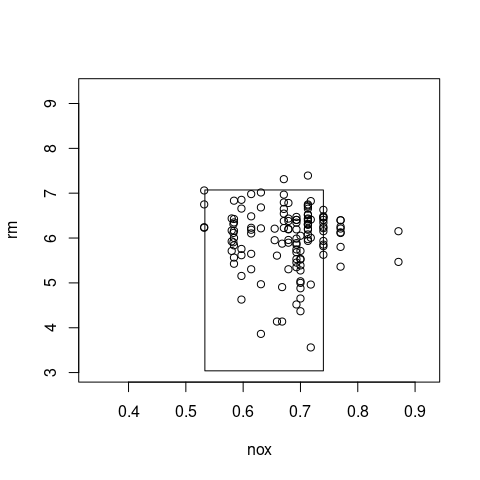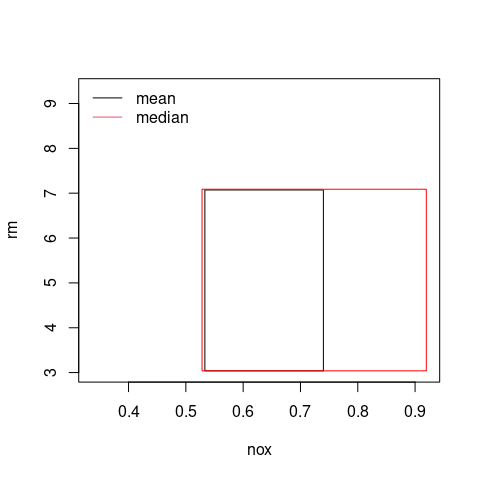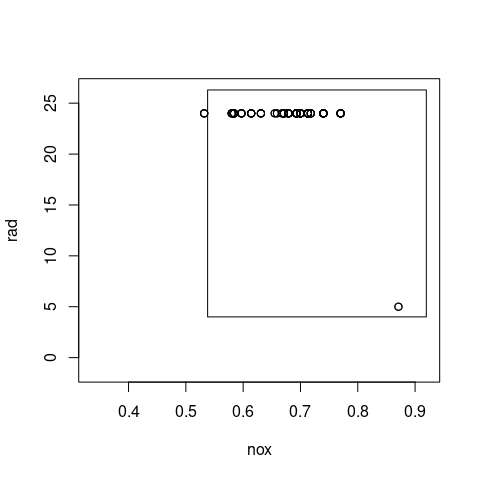# Using prim for bump hunting

#### 04 January 2023

The Patient Rule Induction Method (PRIM) was introduced by Friedman and Fisher (1999). It is a technique from data mining for finding interesting’ regions in high-dimensional data. We start with regression-type data (X1, Y1), …, (Xn, Yn) where Xi is d-dimensional and Yi is a scalar response variable. We are interested in the conditional expectation function

m(x) = E (Y | X = x).

In the case where we have a single sample then PRIM finds the bumps of m(x).

We use a subset of the Boston data set in the MASS library. It contains housing data measurements for 506 towns in the Boston, USA area. For the explanatory variables, we take the nitrogen oxides concentration in parts per 10 million nox and the average number of rooms per dwelling rm. The response is the per capita crime rate crim. We are interested in characterising those areas with higher crime rates in order to provide better support infrastructure.

library(prim)
library(MASS)
data(Boston)
x <- Boston[,5:6]
y <- Boston[,1]
boston.prim <- prim.box(x=x, y=y, threshold.type=1)

The default settings for prim.box are

• peeling quantile: peel.alpha=0.05
• pasting is carried out: pasting=TRUE
• pasting quantile: paste.alpha=0.01
• minimum box mass (proportion of points inside a box): mass.min=0.05
• threshold is the overall mean of the response variable y
• threshold.type=0

We use the default settings except that we wish to only find high crime areas {m(x) $$\ge$$ threshold} so we set threshold.type=1.

We view the output using a summary method. This displays three columns: the box mean, the box mass, and the threshold type. Each line is a summary for each box, as well as an overall summary. The box which is asterisked indicates that it is the box which contains the rest of the data not processed by PRIM. There is one box which contains 42.89% of the towns and where the average crime rate is 7.62. This is our HDR estimate. This regions comprises the bulk of the high crime areas, and is described in terms of nitrogen oxides levels in [0.53, 0.74] and average number of rooms in [3.04, 7.07]. The other 57.11% of the towns have an average crime rate of 0.6035.

summary(boston.prim, print.box=TRUE)
#>           box-fun  box-mass threshold.type
#> box1    7.6222290 0.4288538              1
#> box2*   0.6035267 0.5711462             NA
#> overall 3.6135236 1.0000000             NA
#>
#> Box limits for box1
#>        nox     rm
#> min 0.5332 3.0391
#> max 0.7400 7.0718
#>
#> Box limits for box2
#>        nox     rm
#> min 0.3364 3.0391
#> max 0.9196 9.3019

We plot the PRIM boxes, including all those towns whose crime rate exceeds 3.5. Thus verifying that the majority of high crime towns fall inside thebump.

plot(boston.prim, col="transparent")
points(x[y>3.5,])There are many options for the graphical display. See the help guide for more details ?plot.prim.

The default function applied to the response variable y is mean. We can input another function, e.g. median, using

boston.prim.med <- prim.box(x=x, y=y, threshold.type=1, y.fun=median)

We compare the results: the box for the mean is in black, for the median in red:

plot(boston.prim, col="transparent")
legend("topleft", legend=c("mean", "median"), col=1:2, lty=1, bty="n")The covariate x can also include categorical variables: we replace the average number of rooms per dwelling rm with the index of accessibility to radial highways rad which takes integral values from 1 to 24 inclusive.

x2 <- Boston[,c(5,9)]
y <- Boston[,1]
boston.cat.prim <- prim.box(x=x2, y=y, threshold.type=1)
summary(boston.cat.prim, print.box=TRUE)
#>           box-fun  box-mass threshold.type
#> box1    7.2629703 0.4822134              1
#> box2*   0.2148022 0.5177866             NA
#> overall 3.6135236 1.0000000             NA
#>
#> Box limits for box1
points(x2[y>3.5,])`